# Engineering Mechanics - PKRB: Force and Animation

### Exercise :: PKRB: Force and Animation - General Questions

• PKRB: Force and Animation - General Questions
6.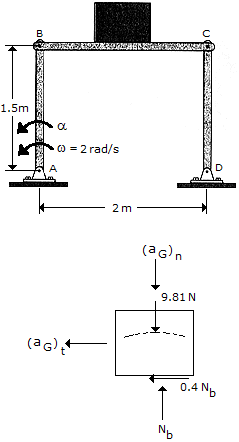The 10-kg block rests on the platform for which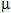= 0.4. If at the instant shown link AB has an angular velocity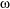= 2 rad/s, determine the greatest angular acceleration of the link so that the block doesn't slip.

 A.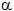= 6.35 rad/s2 B.= 2.62 rad/s2 C.= 1.016 rad/s2 D.= 3.46 rad/s2

Explanation:

No answer description available for this question. Let us discuss.

7.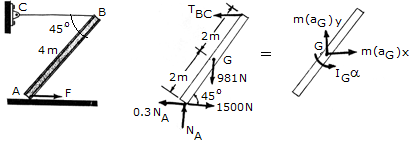If the cable CB is horizontal and the beam is at rest in the position shown, determine the tension in the cable at the instant the towing force F = 1500 N is applied. The coefficient of friction between the beam and the floor at A isA = 0.3. For the calculation, assume that the beam is a uniform slender rod having a mass of 100 kg.

 A. TCB = 636 N B. TCB = 1206 N C. TCB = 1016 N D. TCB = 347 N

Explanation:

No answer description available for this question. Let us discuss.

8.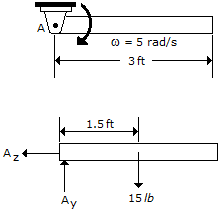The 15-lb rod is pinned and has an angular velocity of= 5 rad/s when it is in the horizontal position shown. Determine the rod's angular acceleration and the pin reactions at this instant.

 A.= 16.1 rad/s2, Ax = 17.47 lb, Ay = 3.75 lb B.= 64.4 rad/s2, Ax = 17.47 lb, Ay = 30.0 lb C.= 16.1 rad/s2, Ax = 17.47 lb, Ay = 15.00 lb D.= 64.4 rad/s2, Ax = 17.47 lb, Ay = 15.00 lb

Explanation:

No answer description available for this question. Let us discuss.

9.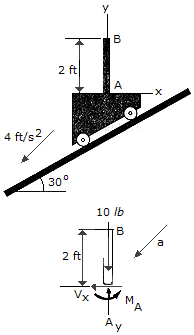Bar AB has a weight of 10 lb and is fixed to the carriage at A. Determine the internal axial force Ay, shear force V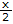, and moment MA at A if the carriage is descending the plane with an acceleration of 4 ft/s2.

 A. Ay = 8.92 lb 8, Vx = 0.621 lb 7, MA = 0 B. Ay = 9.38 lb 8, Vx = 1.076 lb 7, MA = 1.076 lb-ft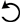C. Ay = 8.92 lb 8, Vx = 0.621 lb 7, MA = 0.621 lb-ftD. Ay = 9.38 lb 8, Vx = 1.076 lb 7, MA = 0

Explanation:

No answer description available for this question. Let us discuss.

10.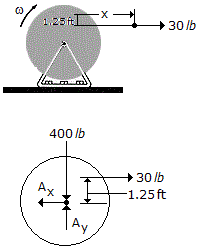A cord wrapped around the inner core of a spool. If the cord is pulled with a constant tension of 30 lb and the spool is originally at rest, determine the spool's angular Velocity when s = 8 ft of cord have unraveled. Neglect the weight of the cord. The spool and cord have a total weight of 400 lb and the radius of gyration about the axle A is kA = 1.30 ft.

 A.= 6.73 rad/s B.= 3.38 rad/s C.= 4.78 rad/s D.= 11.43 rad/s